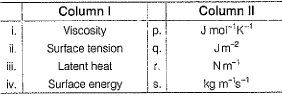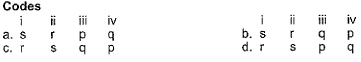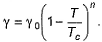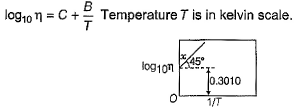Courses

# Test: Viscosity And Surface Tension

## 20 Questions MCQ Test Chemistry for JEE | Test: Viscosity And Surface Tension

Description
This mock test of Test: Viscosity And Surface Tension for JEE helps you for every JEE entrance exam. This contains 20 Multiple Choice Questions for JEE Test: Viscosity And Surface Tension (mcq) to study with solutions a complete question bank. The solved questions answers in this Test: Viscosity And Surface Tension quiz give you a good mix of easy questions and tough questions. JEE students definitely take this Test: Viscosity And Surface Tension exercise for a better result in the exam. You can find other Test: Viscosity And Surface Tension extra questions, long questions & short questions for JEE on EduRev as well by searching above.
QUESTION: 1

### Which of the following properties of water can be used to explain the spherical shape of rain droplets?

Solution:

A simple way to form a drop is to allow liquid to flow slowly from the lower end of a vertical tube of small diameter. The surface tension of the liquid causes the liquid to hang from the tube, forming a pendant. When the drop exceeds a certain size it is no longer stable and detaches itself. The falling liquid is also a drop held together by surface tension.

QUESTION: 2

### How does the surface tension of a liquid vary with increase in temperature?

Solution:

In general, surface tension decreases when temperature increases because cohesive forces decrease with an increase of molecular thermal activity. The influence of the surrounding environment is due to the adhesive action liquid molecules have at the interface.

QUESTION: 3

### Arrange the following in increasing order of surface tension. I (water), II (ethanol), III (hexane)

Solution:

The magnitude of surface tension of liquid depends on the attractive forces between the molecules. In ethanol and water, we have hydrogen bonding. However water has more effective H-bonding owing to its small size. Hexane has induced dipole-induced dipole force which is a weak force. So the order is
III<II<I

QUESTION: 4

Some of the following properties of liquids arise because of the molecular interaction and thermal energy.
I. Vapour pressure II. Surface tension III. Viscosity

Select the properties.

Solution:

Vapour pressure depends on both molecular interaction and thermal energy within molecular attraction vapour pressure decreases and with increase in thermal energy vapour pressure increases.
Surface tension depends on both molecular interaction and thermal energy with increase in molecular interaction surface tension increases.
Viscosity is also depends on Molecular interaction, with increase in molecular attraction viscosity increases.

QUESTION: 5

One of the following properties increase with increase in temperature,

Solution:

Vapor pressure or equilibrium vapor pressure is defined as the pressure exerted by a vapor in thermodynamic equilibrium with its condensed phases at a given temperature in a closed system. The equilibrium vapor pressure is an indication of a liquid's evaporation rate.

QUESTION: 6

Which is equal to 1 kg m-1 S-1?

Solution:

The correct answer is option D
The SI unit of viscosity is the pascal second [Pa s].
The most common unit of viscosity is the dyne second per square centimeter[dyne s/cm2],
Which is given the name poise [P] after the French physiologist Jean Poiseuille
We know that 1 Pa = 1Nm-2 and 1N = 1kgms-2
So, from these relations we can get,
1 Pa-s = 1Nm-2s = 1 kgm-2 s-1
Ten poise equal one pascal second [Pa s] making the centipoise [cP] and millipascal second [mPa s] identical.
Hence, 10 poise = 1 kg m-1 s-1

QUESTION: 7

Surface tension of water is 73 dynes cm -1 at 20° C. If surface area is increased by 0.10 m2, work done is

Solution:

Work done = surface tension×Change in area
Surface tension = 73 dyne cm-1 or 73 x 10-3 N/m
Area = 0.10 m2
So, work done = 73×10-3 x 0.10
= 73 x 10-4 J or 73×10-4 x 107 erg
= 7.3×104 erg

QUESTION: 8

The coefficient of viscosity of a species is 2.10x 10-5 Pa-s. This value is also equal to

Solution:
QUESTION: 9

Direction (Q. Nos. 9) Choice the correct combination of elements and column I and coloumn II  are given as option (a), (b), (c) and (d), out of which ONE option is correct.

Q. Match the physical properties given in Column I with the corresponding units given in Column II and select the correct answer from the codes given below.Solution:

Viscosity = kg m-1 s-1
Surface tension = N m-1
Surface energy = J m-2
For latent heat, we have J kg-1 and not as in p.

*Multiple options can be correct
QUESTION: 10

Direction (Q. Nos. 10-12) This section contains 3 multiple choice questions. Each question has four choices (a), (b), (c) and (d), out of which ONE or  MORE THANT ONE  is correct.

Q. Select correct statement(s).

Solution:

The spherical shape is the shape in which it is most stable due to its surface tension. On earth also it is applicable. A drop of water wants to be in a spherical shape but is affected by other forces, so it becomes distorted. The shape is due to surface tension and not pressure. In space there is no force available to distort the spherical shape.
Adding soaps and detergents that disrupt the intermolecular attractions between adjacent water molecules can reduce the surface tension of water. Because they affect the surface properties of a liquid, soaps, and detergents are called surface-active agents, or surfactants.
Surface tension decreases when the temperature increases because cohesive forces decrease with an increase in molecular thermal activity.

*Multiple options can be correct
QUESTION: 11

Select the correct statement(s).

Solution:

a) True, cohesive forces are intermolecular forces between like molecules and adhesive forces between unlike molecules.
b) True, only due to cohesive force, all the molecules of droplets(which are like molecule) are attracted towards each other to form drop
c) True, if the cohesive force becomes weak as to adhesive forces, then there will be no force to bind water molecules and the drop will collapse and spread into film.
d) True, It's only due to cohesive force that mercury doesn’t wet the glass.

*Multiple options can be correct
QUESTION: 12

The temperature dependence of the surface tension of a liquid is given by the Sugden equation,.

Select correct statement(s).

Solution:
QUESTION: 13

Direction (Q. Nos. 13 and 18) This sectionis based on statement I and Statement II. Select the correct answer from the code given below

Q.

Statement I : Increase in temperature decreases viscosity of liquid.

Statement II : Increase in temperature and thus kinetic energy can overcome intermolecular forces of attraction.

Solution:

Viscosity of liquids decreases as the temperature rises because at high temperature, molecules have high kinetic energy and can overcome the intermolecular forces to slip past one another between the layers.

QUESTION: 14

Statement I : The order of viscosities o f hexane, water and glycerol is hexane < water < glycerol.

Statement II : Hexane has weakest intermolecular forces and glycerol the 1 strongest.

Solution:

In water and glycerine, the intermolecular force that exists is  Hydrogen bonding.
In the case of Hexane, Dispersion forces / London forces exists.
The order of viscosities of these liquids is hexane < water < glycerine.
Hexane has weakest intermolecular forces and glycerine the strongest. ( three OH groups).
Therefore, hexane has minimum viscosity and glycerine has maximum viscosity.

QUESTION: 15

Statement I : Liquids tend to have maximum number of molecules at their surface.

Statement II : Small liquid drops have spherical shape.

Solution:

Liquids try to reduce their surface tension and also the no of molecules at the surface.So statement I is incorrect.
Statement II is correct. Small liquid drops have spherical shape as they try to reduce their surface tension and their surface area. So for a fixed volume, the sphere has the least surface area.

QUESTION: 16

Statement I : As temperature is increased, less work is required to extend the surface of a liquid therefore surface tension decreases.

Statement II : As the temperature and hence the intensity of molecular motion increases, intermolecular forces become less effective.

Solution:

Both statements are true and statement IIis the correct explanation of statement I. As temperature is increased, less work is required to extend the surface of a liquid therefore surface tension decreases. The reason behind is that with increase in temperature, molecular motion increases and so intermolecular forces become less effective.

QUESTION: 17

Statement I : Capillary action is due to surface tension.

Statement II : Mercury, with its strong cohesive forces and weaker adhesive forces, does not show capillary rise.

Solution:
QUESTION: 18

Statement I : A certain amount of energy called activation energy (Ea) is required to move into a hole.

Statement II : On increasing temperature, Ea is available and liquid can flow more easily thus, viscosity decreases.

Solution:
QUESTION: 19

Direction (Q. Nos. 11-13) This section contains a paragraph, wach describing theory, experiments, data etc. three Questions related to paragraph have been given.Each question have only one correct answer among the four given options (a),(b),(c),(d).

Variation of viscosity (q) with temperature T is given byQ. What is viscosity at 100 K?

Solution:

From the graph, we can see that;
log10 ƞ = 0.3010 + 1/T
= 0.3010 + 1/100   (at 100K)   -------------(i)
Given that variation of viscosity(q) = log10ƞ with T
Therefore, log10 ƞ = 0.311
As only for log10 ƞ = 0.311, we will have eqn i correct

QUESTION: 20

Variation of viscosity (q) with temperature T is given byQ. Energy of activation required to move into a hole is

Solution: